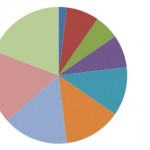# Descriptive Statistics: Definition & Charts and Graphs

Share on

Probability and Statistics > Descriptive Statistics## 1. Definition of Descriptive Statistics

Watch the video for an overview:

Descriptive statistics are one of the fundamental “must knows” with any set of data. It gives you a general idea of trends in your data including:

Descriptive statistics is useful because it allows you to take a large amount of data and summarize it. For example, let’s say you had data on the incomes of one million people. No one is going to want to read a million pieces of data; if they did, they wouldn’t be able to glean any useful information from it. On the other hand, if you summarize it, it becomes useful: an average wage, or a median income, is much easier to understand than reams of data.

## Sub-Areas

Descriptive statistics can be further broken down into several sub-areas, like:

The charts, graphs and plots site index is below. For definitions and information on how to find measures of spread and central tendency, see: Basic statistics (which covers the basic terms you’ll find in descriptive statistics like interquartile range, outliers and standard deviation).

## 2. Difference Between Descriptive and Inferential Statistics

Statistics can be broken down into two areas:

• Descriptive statistics: describes and summarizes data. You are just describing what the data shows: a trend, a specific feature, or a certain statistic (like a mean or median).
• Inferential statistics: uses statistics to make predictions.

Descriptive statistics just describes data. For example, descriptive statistics about a college could include: the average SAT score for incoming freshmen; the median income of parents; racial makeup of the student body. It says nothing about why the data might exist, or what trends you might be able to see from the data. When you take your data and start to make predictions about future behavior or trends, that’s inferential statistics. Inferential statistics also allows you to take sample data (e.g. from one university) and apply it to a larger population (e.g. all universities in the country).

## 3. Excel Descriptive Statistics

Watch the video for the steps:Using the descriptive statistics feature in Excel means that you won’t have to type in individual functions like MEAN or MODE. One button click will return a dozen different stats for your data set. If you want to calculate Excel descriptive statistics, you must have the Data Analysis Toolpak loaded in Excel. Click the “Data” tab in Excel. If you don’t see “Data analysis” on the right of the toolbar, you need to load the Toolpak first. See: Load the Excel Data Analysis Toolpak.

### How to Calculate Excel Descriptive Statistics: Steps

Step 1: Type your data into Excel, in a single column. For example, if you have ten items in your data set, type them into cells A1 through A10.

Step 2: Click the “Data” tab and then click “Data Analysis” in the Analysis group.

Step 3: Highlight “Descriptive Statistics” in the pop-up Data Analysis window.

Step 4: Type an input range into the “Input Range” text box. For this example, type “A1:A10” into the box.

Step 5: Check the “Labels in first row” check box if you have titled the column in row 1, otherwise leave the box unchecked.

Step 6: Type a cell location into the “Output Range” box. For example, type “C1.” Make sure that two adjacent columns do not have data in them.

Step 7: Click the “Summary Statistics” check box and then click “OK” to display Excel descriptive statistics. A list of descriptive statistics will be returned in the column you selected as the Output Range.

## 4. Descriptive Statistics: Charts, Graphs and Plots

There are literally dozens of charts and graphs you can make from data. which one you choose depends upon what kind of data you have and what you want to display. For example, if you wanted to display relationships between data in categories, you could make a bar graph.

A pie chart would show you how categories in your data relate to the whole set.

Scatter plots are a good way to display data points.

Less common, but useful in some cases, include dot plots and box and whisker charts:

## References

Dodge, Y. (2008). The Concise Encyclopedia of Statistics. Springer.
Everitt, B. S.; Skrondal, A. (2010), The Cambridge Dictionary of Statistics, Cambridge University Press.
Gonick, L. (1993). The Cartoon Guide to Statistics. HarperPerennial.
Salkind, N. (2016). Statistics for People Who (Think They) Hate Statistics: Using Microsoft Excel 4th Edition.

CITE THIS AS:
Stephanie Glen. "Descriptive Statistics: Definition & Charts and Graphs" From StatisticsHowTo.com: Elementary Statistics for the rest of us! https://www.statisticshowto.com/probability-and-statistics/descriptive-statistics/
---------------------------------------------------------------------------Need help with a homework or test question? With Chegg Study, you can get step-by-step solutions to your questions from an expert in the field. Your first 30 minutes with a Chegg tutor is free!